# 12.4 Rotation of axes  (Page 5/8)

 Page 5 / 8

Identify the conic for each of the following without rotating axes.

1. ${x}^{2}-9xy+3{y}^{2}-12=0$
2. $10{x}^{2}-9xy+4{y}^{2}-4=0$
1. hyperbola
2. ellipse

Access this online resource for additional instruction and practice with conic sections and rotation of axes.

## Key equations

 General Form equation of a conic section $A{x}^{2}+Bxy+C{y}^{2}+Dx+Ey+F=0$ Rotation of a conic section Angle of rotation

## Key concepts

• Four basic shapes can result from the intersection of a plane with a pair of right circular cones connected tail to tail. They include an ellipse, a circle, a hyperbola, and a parabola.
• A nondegenerate conic section has the general form $\text{\hspace{0.17em}}A{x}^{2}+Bxy+C{y}^{2}+Dx+Ey+F=0\text{\hspace{0.17em}}$ where $\text{\hspace{0.17em}}A,B\text{\hspace{0.17em}}$ and $\text{\hspace{0.17em}}C\text{\hspace{0.17em}}$ are not all zero. The values of $\text{\hspace{0.17em}}A,B,$ and $\text{\hspace{0.17em}}C\text{\hspace{0.17em}}$ determine the type of conic. See [link] .
• Equations of conic sections with an $\text{\hspace{0.17em}}xy\text{\hspace{0.17em}}$ term have been rotated about the origin. See [link] .
• The general form can be transformed into an equation in the $\text{\hspace{0.17em}}{x}^{\prime }\text{\hspace{0.17em}}$ and $\text{\hspace{0.17em}}{y}^{\prime }\text{\hspace{0.17em}}$ coordinate system without the $\text{\hspace{0.17em}}{x}^{\prime }{y}^{\prime }\text{\hspace{0.17em}}$ term. See [link] and [link] .
• An expression is described as invariant if it remains unchanged after rotating. Because the discriminant is invariant, observing it enables us to identify the conic section. See [link] .

## Verbal

What effect does the $\text{\hspace{0.17em}}xy\text{\hspace{0.17em}}$ term have on the graph of a conic section?

The $\text{\hspace{0.17em}}xy\text{\hspace{0.17em}}$ term causes a rotation of the graph to occur.

If the equation of a conic section is written in the form $\text{\hspace{0.17em}}A{x}^{2}+B{y}^{2}+Cx+Dy+E=0\text{\hspace{0.17em}}$ and $\text{\hspace{0.17em}}AB=0,$ what can we conclude?

If the equation of a conic section is written in the form $\text{\hspace{0.17em}}A{x}^{2}+Bxy+C{y}^{2}+Dx+Ey+F=0,$ and $\text{\hspace{0.17em}}{B}^{2}-4AC>0,$ what can we conclude?

The conic section is a hyperbola.

Given the equation $\text{\hspace{0.17em}}a{x}^{2}+4x+3{y}^{2}-12=0,$ what can we conclude if $\text{\hspace{0.17em}}a>0?$

For the equation $\text{\hspace{0.17em}}A{x}^{2}+Bxy+C{y}^{2}+Dx+Ey+F=0,$ the value of $\text{\hspace{0.17em}}\theta \text{\hspace{0.17em}}$ that satisfies $\text{\hspace{0.17em}}\mathrm{cot}\left(2\theta \right)=\frac{A-C}{B}\text{\hspace{0.17em}}$ gives us what information?

It gives the angle of rotation of the axes in order to eliminate the $\text{\hspace{0.17em}}xy\text{\hspace{0.17em}}$ term.

## Algebraic

For the following exercises, determine which conic section is represented based on the given equation.

$9{x}^{2}+4{y}^{2}+72x+36y-500=0$

${x}^{2}-10x+4y-10=0$

$AB=0,$ parabola

$2{x}^{2}-2{y}^{2}+4x-6y-2=0$

$4{x}^{2}-{y}^{2}+8x-1=0$

$AB=-4<0,$ hyperbola

$4{y}^{2}-5x+9y+1=0$

$2{x}^{2}+3{y}^{2}-8x-12y+2=0$

$AB=6>0,$ ellipse

$4{x}^{2}+9xy+4{y}^{2}-36y-125=0$

$3{x}^{2}+6xy+3{y}^{2}-36y-125=0$

${B}^{2}-4AC=0,$ parabola

$-3{x}^{2}+3\sqrt{3}xy-4{y}^{2}+9=0$

$2{x}^{2}+4\sqrt{3}xy+6{y}^{2}-6x-3=0$

${B}^{2}-4AC=0,$ parabola

$-{x}^{2}+4\sqrt{2}xy+2{y}^{2}-2y+1=0$

$8{x}^{2}+4\sqrt{2}xy+4{y}^{2}-10x+1=0$

${B}^{2}-4AC=-96<0,$ ellipse

For the following exercises, find a new representation of the given equation after rotating through the given angle.

$3{x}^{2}+xy+3{y}^{2}-5=0,\theta =45°$

$4{x}^{2}-xy+4{y}^{2}-2=0,\theta =45°$

$7{{x}^{\prime }}^{2}+9{{y}^{\prime }}^{2}-4=0$

$2{x}^{2}+8xy-1=0,\theta =30°$

$-2{x}^{2}+8xy+1=0,\theta =45°$

$3{{x}^{\prime }}^{2}+2{x}^{\prime }{y}^{\prime }-5{{y}^{\prime }}^{2}+1=0$

$4{x}^{2}+\sqrt{2}xy+4{y}^{2}+y+2=0,\theta =45°$

For the following exercises, determine the angle $\text{\hspace{0.17em}}\theta \text{\hspace{0.17em}}$ that will eliminate the $\text{\hspace{0.17em}}xy\text{\hspace{0.17em}}$ term and write the corresponding equation without the $\text{\hspace{0.17em}}xy\text{\hspace{0.17em}}$ term.

${x}^{2}+3\sqrt{3}xy+4{y}^{2}+y-2=0$

$\theta ={60}^{\circ },11{{x}^{\prime }}^{2}-{{y}^{\prime }}^{2}+\sqrt{3}{x}^{\prime }+{y}^{\prime }-4=0$

$4{x}^{2}+2\sqrt{3}xy+6{y}^{2}+y-2=0$

$9{x}^{2}-3\sqrt{3}xy+6{y}^{2}+4y-3=0$

$\theta ={150}^{\circ },21{{x}^{\prime }}^{2}+9{{y}^{\prime }}^{2}+4{x}^{\prime }-4\sqrt{3}{y}^{\prime }-6=0$

$-3{x}^{2}-\sqrt{3}xy-2{y}^{2}-x=0$

$16{x}^{2}+24xy+9{y}^{2}+6x-6y+2=0$

$\theta \approx {36.9}^{\circ },125{{x}^{\prime }}^{2}+6{x}^{\prime }-42{y}^{\prime }+10=0$

${x}^{2}+4xy+4{y}^{2}+3x-2=0$

${x}^{2}+4xy+{y}^{2}-2x+1=0$

$\theta ={45}^{\circ },3{{x}^{\prime }}^{2}-{{y}^{\prime }}^{2}-\sqrt{2}{x}^{\prime }+\sqrt{2}{y}^{\prime }+1=0$

$4{x}^{2}-2\sqrt{3}xy+6{y}^{2}-1=0$

## Graphical

For the following exercises, rotate through the given angle based on the given equation. Give the new equation and graph the original and rotated equation.

$y=-{x}^{2},\theta =-{45}^{\circ }$

$\frac{\sqrt{2}}{2}\left({x}^{\prime }+{y}^{\prime }\right)=\frac{1}{2}{\left({x}^{\prime }-{y}^{\prime }\right)}^{2}$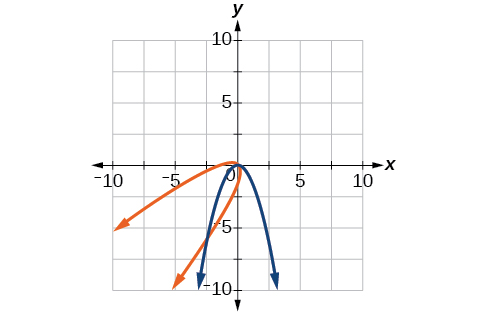$x={y}^{2},\theta ={45}^{\circ }$

$\frac{{x}^{2}}{4}+\frac{{y}^{2}}{1}=1,\theta ={45}^{\circ }$

$\frac{{\left({x}^{\prime }-{y}^{\prime }\right)}^{2}}{8}+\frac{{\left({x}^{\prime }+{y}^{\prime }\right)}^{2}}{2}=1$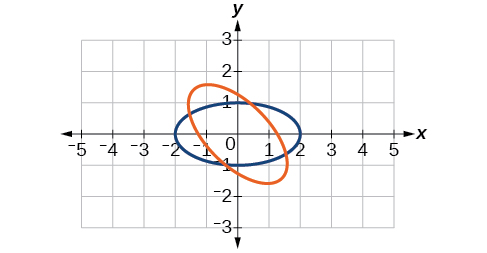$\frac{{y}^{2}}{16}+\frac{{x}^{2}}{9}=1,\theta ={45}^{\circ }$

${y}^{2}-{x}^{2}=1,\theta ={45}^{\circ }$

$\frac{{\left({x}^{\prime }+{y}^{\prime }\right)}^{2}}{2}-\frac{{\left({x}^{\prime }-{y}^{\prime }\right)}^{2}}{2}=1$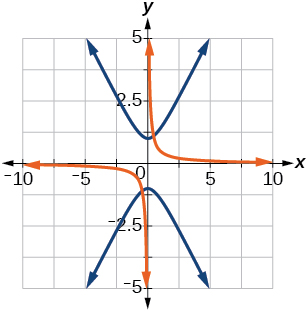$y=\frac{{x}^{2}}{2},\theta ={30}^{\circ }$

$x={\left(y-1\right)}^{2},\theta ={30}^{\circ }$

$\frac{\sqrt{3}}{2}{x}^{\prime }-\frac{1}{2}{y}^{\prime }={\left(\frac{1}{2}{x}^{\prime }+\frac{\sqrt{3}}{2}{y}^{\prime }-1\right)}^{2}$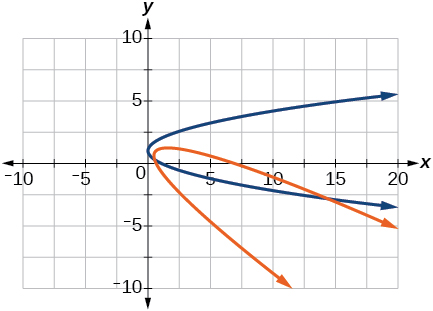$\frac{{x}^{2}}{9}+\frac{{y}^{2}}{4}=1,\theta ={30}^{\circ }$

For the following exercises, graph the equation relative to the $\text{\hspace{0.17em}}{x}^{\prime }{y}^{\prime }\text{\hspace{0.17em}}$ system in which the equation has no $\text{\hspace{0.17em}}{x}^{\prime }{y}^{\prime }\text{\hspace{0.17em}}$ term.

$xy=9$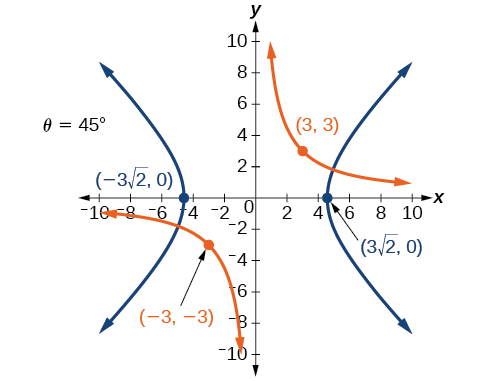${x}^{2}+10xy+{y}^{2}-6=0$

${x}^{2}-10xy+{y}^{2}-24=0$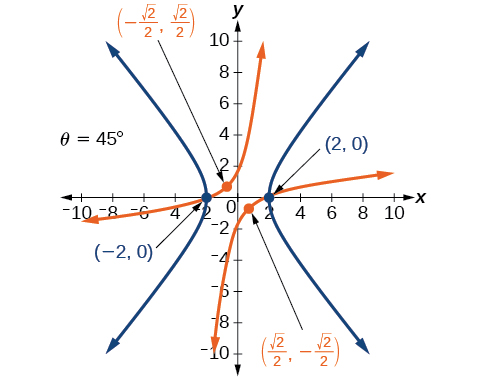$4{x}^{2}-3\sqrt{3}xy+{y}^{2}-22=0$

$6{x}^{2}+2\sqrt{3}xy+4{y}^{2}-21=0$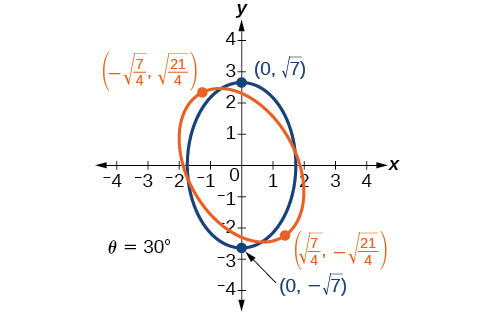$11{x}^{2}+10\sqrt{3}xy+{y}^{2}-64=0$

$21{x}^{2}+2\sqrt{3}xy+19{y}^{2}-18=0$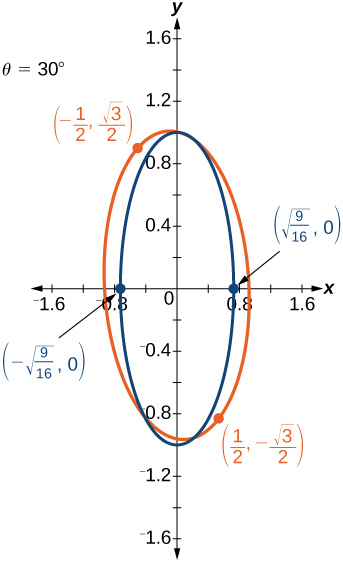$16{x}^{2}+24xy+9{y}^{2}-130x+90y=0$

$16{x}^{2}+24xy+9{y}^{2}-60x+80y=0$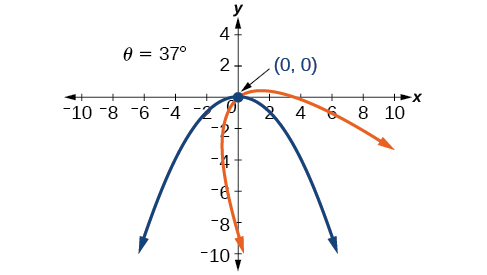$13{x}^{2}-6\sqrt{3}xy+7{y}^{2}-16=0$

$4{x}^{2}-4xy+{y}^{2}-8\sqrt{5}x-16\sqrt{5}y=0$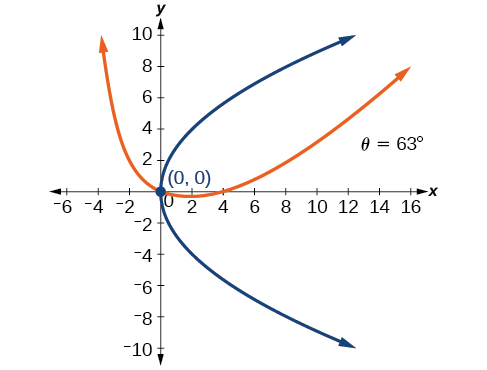For the following exercises, determine the angle of rotation in order to eliminate the $\text{\hspace{0.17em}}xy\text{\hspace{0.17em}}$ term. Then graph the new set of axes.

$6{x}^{2}-5\sqrt{3}xy+{y}^{2}+10x-12y=0$

$6{x}^{2}-5xy+6{y}^{2}+20x-y=0$

$\theta ={45}^{\circ }$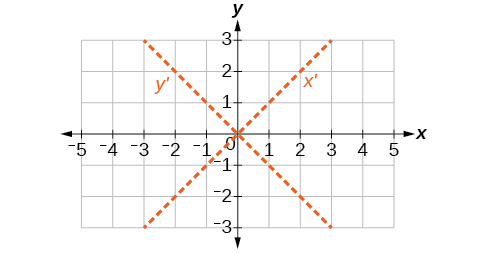$6{x}^{2}-8\sqrt{3}xy+14{y}^{2}+10x-3y=0$

$4{x}^{2}+6\sqrt{3}xy+10{y}^{2}+20x-40y=0$

$\theta ={60}^{\circ }$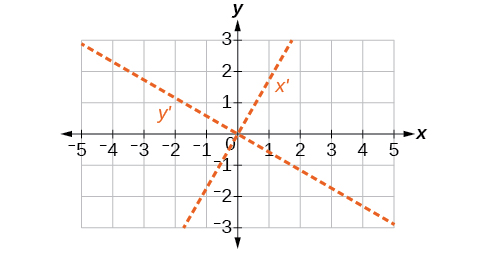$8{x}^{2}+3xy+4{y}^{2}+2x-4=0$

$16{x}^{2}+24xy+9{y}^{2}+20x-44y=0$

$\theta \approx {36.9}^{\circ }$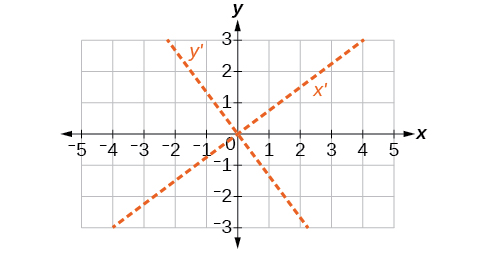For the following exercises, determine the value of $\text{\hspace{0.17em}}k\text{\hspace{0.17em}}$ based on the given equation.

Given $\text{\hspace{0.17em}}4{x}^{2}+kxy+16{y}^{2}+8x+24y-48=0,$ find $\text{\hspace{0.17em}}k\text{\hspace{0.17em}}$ for the graph to be a parabola.

Given $\text{\hspace{0.17em}}2{x}^{2}+kxy+12{y}^{2}+10x-16y+28=0,$ find $\text{\hspace{0.17em}}k\text{\hspace{0.17em}}$ for the graph to be an ellipse.

$-4\sqrt{6}

Given $\text{\hspace{0.17em}}3{x}^{2}+kxy+4{y}^{2}-6x+20y+128=0,$ find $\text{\hspace{0.17em}}k\text{\hspace{0.17em}}$ for the graph to be a hyperbola.

Given $\text{\hspace{0.17em}}k{x}^{2}+8xy+8{y}^{2}-12x+16y+18=0,$ find $\text{\hspace{0.17em}}k\text{\hspace{0.17em}}$ for the graph to be a parabola.

$k=2$

Given $\text{\hspace{0.17em}}6{x}^{2}+12xy+k{y}^{2}+16x+10y+4=0,$ find $\text{\hspace{0.17em}}k\text{\hspace{0.17em}}$ for the graph to be an ellipse.

what are you up to?
nothing up todat yet
Miranda
hi
jai
hello
jai
Miranda Drice
jai
aap konsi country se ho
jai
which language is that
Miranda
I am living in india
jai
good
Miranda
what is the formula for calculating algebraic
I think the formula for calculating algebraic is the statement of the equality of two expression stimulate by a set of addition, multiplication, soustraction, division, raising to a power and extraction of Root. U believe by having those in the equation you will be in measure to calculate it
Miranda
state and prove Cayley hamilton therom
hello
Propessor
hi
Miranda
the Cayley hamilton Theorem state if A is a square matrix and if f(x) is its characterics polynomial then f(x)=0 in another ways evey square matrix is a root of its chatacteristics polynomial.
Miranda
hi
jai
hi Miranda
jai
thanks
Propessor
welcome
jai
What is algebra
algebra is a branch of the mathematics to calculate expressions follow.
Miranda
Miranda Drice would you mind teaching me mathematics? I think you are really good at math. I'm not good at it. In fact I hate it. 😅😅😅
Jeffrey
lolll who told you I'm good at it
Miranda
something seems to wispher me to my ear that u are good at it. lol
Jeffrey
lolllll if you say so
Miranda
but seriously, Im really bad at math. And I hate it. But you see, I downloaded this app two months ago hoping to master it.
Jeffrey
which grade are you in though
Miranda
oh woww I understand
Miranda
Jeffrey
Jeffrey
Miranda
how come you finished in college and you don't like math though
Miranda
gotta practice, holmie
Steve
if you never use it you won't be able to appreciate it
Steve
I don't know why. But Im trying to like it.
Jeffrey
yes steve. you're right
Jeffrey
so you better
Miranda
what is the solution of the given equation?
which equation
Miranda
I dont know. lol
Jeffrey
Miranda
Jeffrey
answer and questions in exercise 11.2 sums
how do u calculate inequality of irrational number?
Alaba
give me an example
Chris
and I will walk you through it
Chris
cos (-z)= cos z .
cos(- z)=cos z
Mustafa
what is a algebra
(x+x)3=?
6x
Obed
what is the identity of 1-cos²5x equal to?
__john __05
Kishu
Hi
Abdel
hi
Ye
hi
Nokwanda
C'est comment
Abdel
Hi
Amanda
hello
SORIE
Hiiii
Chinni
hello
Ranjay
hi
ANSHU
hiiii
Chinni
h r u friends
Chinni
yes
Hassan
so is their any Genius in mathematics here let chat guys and get to know each other's
SORIE
I speak French
Abdel
okay no problem since we gather here and get to know each other
SORIE
hi im stupid at math and just wanna join here
Yaona
lol nahhh none of us here are stupid it's just that we have Fast, Medium, and slow learner bro but we all going to work things out together
SORIE
it's 12
what is the function of sine with respect of cosine , graphically
tangent bruh
Steve
cosx.cos2x.cos4x.cos8x
sinx sin2x is linearly dependent
what is a reciprocal
The reciprocal of a number is 1 divided by a number. eg the reciprocal of 10 is 1/10 which is 0.1
Shemmy
Reciprocal is a pair of numbers that, when multiplied together, equal to 1. Example; the reciprocal of 3 is ⅓, because 3 multiplied by ⅓ is equal to 1
Jeza
each term in a sequence below is five times the previous term what is the eighth term in the sequence
I don't understand how radicals works pls
How look for the general solution of a trig functionByByBy Richley CrapoBy OpenStaxBy OpenStaxBy Sarah WarrenBy Jams KaloBy OpenStaxBy Michael SagBy Richley CrapoBy Madison ChristianBy Michael Pitt